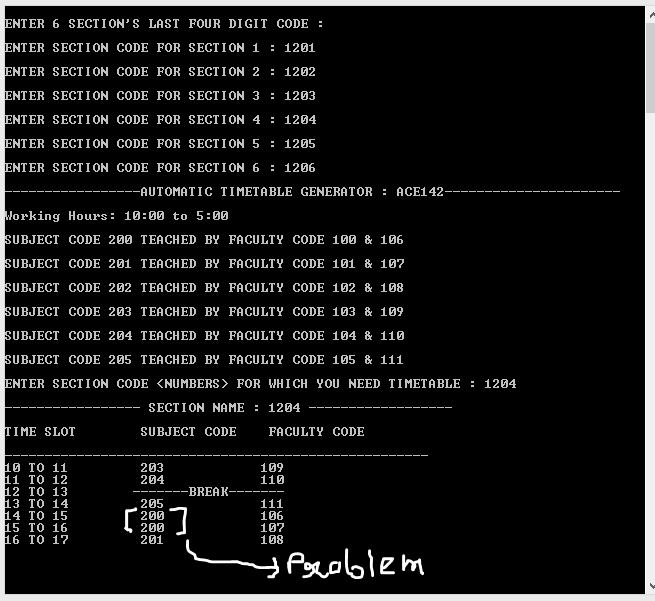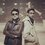# Debug my program : Solution unknownI got a problem in which i have to assign 6 subject and 12 teachers to 6 sections on condition where -

Every class should study different subject for particular hour.

Lunch will be between 12-3, and each section is provided with 1 hr gap.

No teacher can take more than three lecture in a day

I designed the solution but after certain value of subject it repeats the subject, while the teacher code is work perfectly Can you find the bug -

// * SECTION ASSIGNING FUNCTION *

int timetable::assign() {

int i=0,j,k,c;
if(cop==0)                                                                       // IF SECTION ==NULL
{
section[i].name=sec_code[i];
for(j=i,k=0;k<6 && sub_code[j]<=205;j++,k++)                     // CONDITION1
{
section[i].subject[k]=sub_code[j];
if(fac_code[j].coun<3)                    // CONDITION 3
{
section[i].faculty[k]=fac_code[j].fac_cod;
fac_code[j].coun++;
}
else
{
section[i].faculty[k]=fac_code[j+6].fac_cod;
fac_code[j+6].coun++;
}
}
cop=1;
}                                                                               //for second loop;
else
{
for(int i=1;i<6;i++)
{
section[i].name=sec_code[i];
for(int j=i,k=0;k<6;j++,k++)
{
section[i].subject[k]=sub_code[j];                  // problem is here
if(fac_code[j].coun<3)
{
section[i].faculty[k]=fac_code[j].fac_cod;
fac_code[j].coun++;
}
else
{
section[i].faculty[k]=fac_code[j+6].fac_cod;
fac_code[j+6].coun++;
}
}
}                                                                            //break IF >205 ::: CONDITION 2
for(int i=1;i<6;i++)
{
for(int j=0,k=0;k<6;k++)
{
if(section[i].subject[k]<=205 && section[i].subject[k]>=200)
{
}
else
{
section[i].subject[k]=sub_code[j];
if(fac_code[j].coun<3)                              //IF FACULTY ASSIGNED TO MORE THAN THREE
{
section[i].faculty[k]=fac_code[j].fac_cod;
fac_code[j].coun++;
}
else
{
section[i].faculty[k]=fac_code[j+6].fac_cod;
fac_code[j+6].coun++;
}
j++;
}
}
}
}


}

//THE DISPLAY TERMINAL CONTAINS THE LUNCH PARTNote by Tanmay Baranwal
7 years, 3 months ago

This discussion board is a place to discuss our Daily Challenges and the math and science related to those challenges. Explanations are more than just a solution — they should explain the steps and thinking strategies that you used to obtain the solution. Comments should further the discussion of math and science.

When posting on Brilliant:

• Use the emojis to react to an explanation, whether you're congratulating a job well done , or just really confused .
• Ask specific questions about the challenge or the steps in somebody's explanation. Well-posed questions can add a lot to the discussion, but posting "I don't understand!" doesn't help anyone.
• Try to contribute something new to the discussion, whether it is an extension, generalization or other idea related to the challenge.

MarkdownAppears as
*italics* or _italics_ italics
**bold** or __bold__ bold
- bulleted- list
• bulleted
• list
1. numbered2. list
1. numbered
2. list
Note: you must add a full line of space before and after lists for them to show up correctly
paragraph 1paragraph 2

paragraph 1

paragraph 2

[example link](https://brilliant.org)example link
> This is a quote
This is a quote
    # I indented these lines
# 4 spaces, and now they show
# up as a code block.

print "hello world"
# I indented these lines
# 4 spaces, and now they show
# up as a code block.

print "hello world"
MathAppears as
Remember to wrap math in $$ ... $$ or $ ... $ to ensure proper formatting.
2 \times 3 $2 \times 3$
2^{34} $2^{34}$
a_{i-1} $a_{i-1}$
\frac{2}{3} $\frac{2}{3}$
\sqrt{2} $\sqrt{2}$
\sum_{i=1}^3 $\sum_{i=1}^3$
\sin \theta $\sin \theta$
\boxed{123} $\boxed{123}$